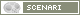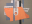OpenMusic DocumentationOM 6.6 User Manual > Lisp Programming > Introduction to Lisp
page précédentepage suivante

Introduction to Lisp

This section is not a Common Lisp tutorial, but just expounds a few important notions.

Origins

A Bit of History

Lisp is a functional language influenced by the lambda calculus that was specified in 1958. It is one of the oldest high-level programming languages.

Common Lisp is a Lisp dialect, among others such as Scheme .

An Interpreted Language

Lisp is an "interpreted" language : it works on top of a low-level process, or underlying interpreter, which evaluates Lisp code. Functions and data are defined and evaluated by this process, which incrementally enriches the running environment with new functionalities. Each time a new function, variable or class is defined, it becomes part of the environment. This characteristics makes Lisp programming very flexible and dynamic, since code can still be interpreted while Lisp programs are running.

For a more complete overview of the language, the reader should consult specialized books or references. Here are some links of interest :

S-expressions

S-expression stands for "symbolic expression". S-expressions are list-based representations. They are typically represented in text by parenthesized, whitespace-separated sequences of character strings.

Prefix Notation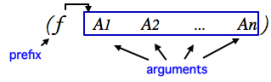Lisp expressions are written as lists with a prefix notation. The first element in the list is an operator or a function, while the remaining elements are treated as data : they are the arguments of this operator.

When an expression is evaluated, the first element is applied to the arguments. For instance :

• An addition such as 1 + 2 + 3 is expressed (+ 1 2 3) , which evaluates to : 6. The + function is applied to 1 2 and 3.

• In the (list 1 2 3) expression, the list operator creates a list with the other elements in the expression, which evaluates to : (1 2 3)

Quoted Expressions : QUOTE Operator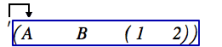The QUOTE operator " ' " allows to get a list from a lisp expression without evaluating it. This operator must be put before the targetted list first parenthesis, and applies to all the elements located between the pair of parenthesis.

For instance :

When the following expression : '(+ 5 6) is evaluated, (+ 5 6) is returned. The list is merely quoted.When the following expression (+ 5 6) is evaluated, 11 is returned. The list is evaluated, because there is no quote.

Lambda Expressions : the LAMBDA Operator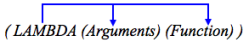The LAMBDA operator binds – associates – variables to values in an expression. In other words, it allows to define functions.

The syntax of a lambda expression is : (lambda (arg1 arg2 ...) [body of the function] ) . This expression evaluates to a function, which can be applied to arguments. At application time, these arguments are bound to variables represented by arg1 , arg2 , ...

For instance, the lambda expression that defines (4 + (a * b)) with a and b as variables is written :

(lambda (a b) (+ 4 (* a b))) .

Functions

Defining Functions : DEFUN

Most Lisp programming consists into defining functions. DEFUN is a particular operator that defines a function bound to a name  :

(defun my-function-name (arg1 arg2 arg3)

[ Lisp expression(s) ]

result )

Once the function is defined, its name represents the function.

Defining Local Variables within a Function : LET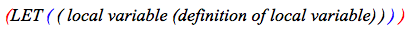LET is a special operator that "declares" local variables in the body of the function. The declaration and the content of the declaration must both be delineated by parenthesis.

For instance, the following expression declares an x local variable :

(let (( x (+ a 5)) ) means : x is a local variable, such as x = a + 5.

Example : Defining My-Function
 Let's define "my-function". (defun my-function (a b c) (let ((x (+ a 5))) (* x (/ b c)))) We can also write it : (defun my-function (a b c)      (let ((x (+ a 5)))           (* x (/ b c))       ) )
 A , b and c are the arguments of the function, the variables that are bound to values, when the function is called . We also have a local variable : (x = a + 5) .The last expression is the result returned by the function itself : ( x * (b / c )).In other words, the expression means : " my-function applies to a , b and c . Besides x = ( a + 5). This function is : x * (b / c) ." My-function is called when its name followed by the relevant set of arguments is evaluated : (my-function 1 2 3) => 4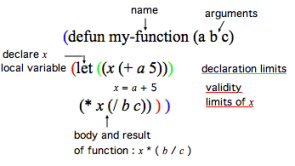1. Argument

An argument represents a parameter upon which a function operates. For instance, the (om+ x y) function has two arguments : x and y.

2. Evaluation

In Lisp, evaluating an expression amounts to interpreting and reducing its contents in order to return a result.

In OM, visual programs represent Lisp expressions and evaluating a box triggers the calculus that will determine the value of these expressions.

Any kind of box can be evaluated and return something. To evaluate a box, ⤷ Click and press v or Cmd click on an outlet.

page précédentepage suivante
A propos...(c) Ircam - Centre Pompidou